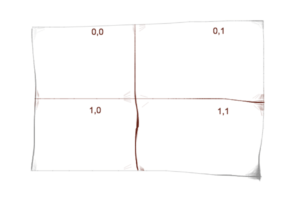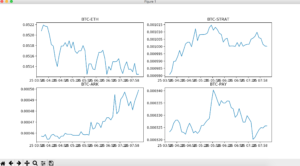In this post I am going to discuss a Matplotlib feature which let you add multiple plots within a figure called subplots.

Subplots are helpful when you want to show different data presentation in a single view, for instance Dashboards.

There are multiple ways you can create subplots but I am here going to discuss the one which let you add graphs in grids by using `subplot2grid` method. `subplot2grid` takes two mandatory parameters, the first one is size and the next is location. A typical `subplot2grid` call will look like below:

`ax = plt.subplot2grid((2, 2), (0, 0))`

The first parameter is the size that is a 2 x 2 grid, the 2nd is the location or quadrant where the graph will be plotted. The illustration will help you to understand it further.So first graph in `0,0`, 2nd in `0,1` and so on..

Now let’s get into code.  The data I am going to use is the Crypto Trading Data of my favorite coins on Bittrex Exchange. We will fetch data latest 5-min interval data from bittrex for Ethereum, Stratis, Ark and TenX coins and display as subplots within a figure.

```import json
import time

import matplotlib.pyplot as plt
import pandas as pd

if __name__ == '__main__':
# ETH
data_eth_btc = get_data('BTC-ETH', 'fivemin')
last_few = data_eth_btc.tail(50)
close_eth = last_few['Close']
last_few['Time'] = pd.to_datetime(last_few['T'])
del last_few['T']

# STRAT
data_strat_btc = get_data('BTC-STRAT', 'fivemin')
last_few_strat = data_strat_btc.tail(50)
close_strat = last_few_strat['Close']
last_few_strat['Time'] = pd.to_datetime(last_few_strat['T'])
del last_few_strat['T']

# ARK
data_ark_btc = get_data('BTC-ARK', 'fivemin')
last_few_ark = data_ark_btc.tail(50)
close_ark = last_few_ark['Close']
last_few_ark['Time'] = pd.to_datetime(last_few_ark['T'])
del last_few_ark['T']

# PAY
data_pay_btc = get_data('BTC-PAY', 'fivemin')
last_few_pay = data_pay_btc.tail(50)
close_pay = last_few_pay['Close']
last_few_pay['Time'] = pd.to_datetime(last_few_pay['T'])
del last_few_pay['T']```

I am using a custom `get_data()` function which takes interval and crypto pair and returns data in Pandas dataframe format. Since the objective is not to teach how data being fetched so I am not giving `get_data()` code for sake of simplicity.

OK data is fetched in dataframe and I am picking last 50 entries thus using `tail()` here. In order to show time data we need to use `pd.to_datetime` to convert in internal datetime format and delete the original time data provided by the API by calling `del` function.

Our required data is available and now we have to plot 4 graphs for different coins.

```    ax = plt.subplot2grid((2, 2), (0, 0))
ax.plot(last_few['Time'], close_eth)
plt.title('BTC-ETH')

ax1 = plt.subplot2grid((2, 2), (0, 1))
ax1.plot(last_few['Time'], close_strat)
plt.title('BTC-STRAT')

ax2 = plt.subplot2grid((2, 2), (1, 0))
ax2.plot(last_few_ark['Time'], close_ark)
plt.title('BTC-ARK')

ax3 = plt.subplot2grid((2, 2), (1, 1))
ax3.plot(last_few_pay['Time'], close_pay)
plt.title('BTC-PAY')

plt.show()```

First, the grid was set and location is set and assigned to `ax` variable and then the graph was plotted. Once all 4 graphs plotted they were then displayed by calling `show()` once and something like below appears:The graphs were plotted as of Dec, 25,2017 around 1PM(GMT+5) and you can see only ARK is on uptrend.

Alright and this sums up the Python Graph series in matplotlib. I might cover the candlestick graph but can’t commit so consider this series done here. As always, do give your feedback in comments below.

## If you like this post then you should subscribe to my blog for future updates.

* indicates required